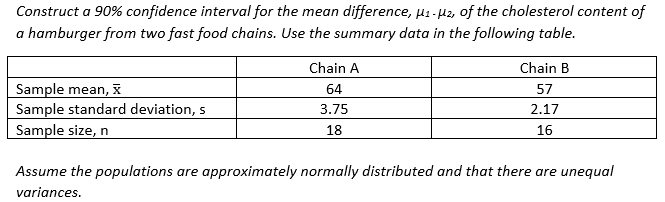# Confidence Interval for Difference between Means using StatCrunch

Similar to MSL problems 8.1.28-T, 8.2.24, and 8.2.25

Although we are rarely given summary data to analyze in the real business world, we often are in statistics courses. Consider the following problem:Further, you are given this frighteningly complex formula to use for finding the CI when the population variances are not equal:Or the z version:And you are told that the degrees of freedom to use to find the critical value of t is the smaller of n1-1 or n2-1.

So, yes, you can jump in with your calculator and solve the equation. Then, use the tables or your graphing calculator to find the critical value of t.

Or, you can use StatCrunch®.

Open StatCrunch and select Stat > T Stats > Two Sample > With Summary.

But wait. How do I decide whether to use the Z or the T?

In this problem, it is trivial since they give you the formula using t (or if they give the version with the z critical).

But, you should carefully read the problem statement looking to see if the population sigmas are given. If they are, use the z critical values.

If the sigmas are not given, look at the sample sizes. Here, the n’s are both less than 30, so that is a strong clue to use the t statistic. The statement the “variances are unequal” is another clue to use the t.

In the dialog box that opens, enter your data for the two samples. Because the variances are not equal, be sure to uncheck the Pool variances box under Calculation Options. Also, be sure to select the radio button next to Confidence interval for µ1 – µ2, and enter the desired confidence level. Then click Compute!The output box gives the upper and lower limits (highlighted in green here) of the confidence interval for the mean difference.

That’s all there is to it.

And remember you can easily switch back and run the hypothesis test by clicking on the Options button in the top left of the Output box: Options > Edit. Then, select the Hypothesis test for µ1 – µ2, and again click Compute!This site uses Akismet to reduce spam. Learn how your comment data is processed.